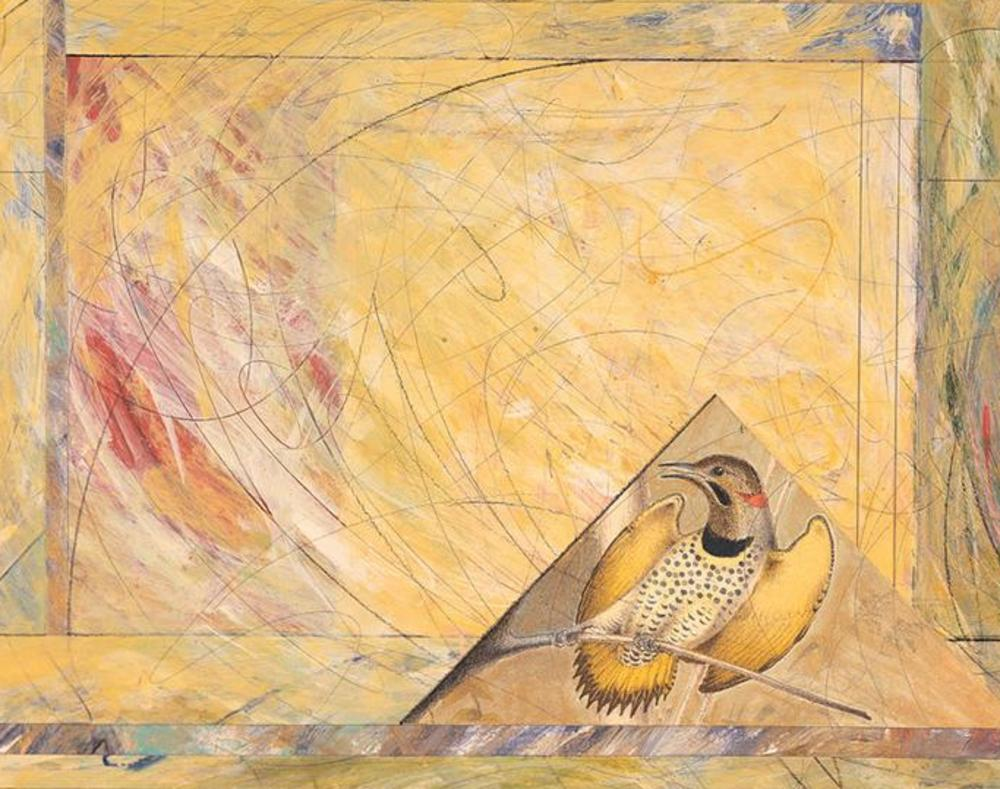M  A  R  T  H  A    A  N  D  R  E  A
F  I  N  E    A  R  T
 H O M EA R T I S T  -  S T A T E M E N TG A L L E R YB I O G R A P H YE X H I B I T I O N S